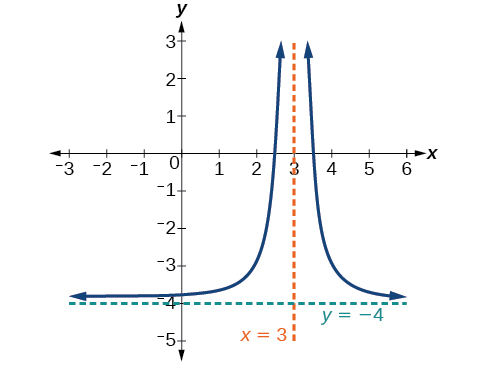5.6 Rational functions  (Page 2/16)

 Page 2 / 16

Vertical asymptote

A vertical asymptote    of a graph is a vertical line $\text{\hspace{0.17em}}x=a\text{\hspace{0.17em}}$ where the graph tends toward positive or negative infinity as the inputs approach $\text{\hspace{0.17em}}a.\text{\hspace{0.17em}}$ We write

End behavior of $\text{\hspace{0.17em}}f\left(x\right)=\frac{1}{x}$

As the values of $\text{\hspace{0.17em}}x\text{\hspace{0.17em}}$ approach infinity, the function values approach 0. As the values of $\text{\hspace{0.17em}}x\text{\hspace{0.17em}}$ approach negative infinity, the function values approach 0. See [link] . Symbolically, using arrow notation

Based on this overall behavior and the graph, we can see that the function approaches 0 but never actually reaches 0; it seems to level off as the inputs become large. This behavior creates a horizontal asymptote , a horizontal line that the graph approaches as the input increases or decreases without bound. In this case, the graph is approaching the horizontal line $\text{\hspace{0.17em}}y=0.\text{\hspace{0.17em}}$ See [link] .

Horizontal asymptote

A horizontal asymptote    of a graph is a horizontal line $\text{\hspace{0.17em}}y=b\text{\hspace{0.17em}}$ where the graph approaches the line as the inputs increase or decrease without bound. We write

Using arrow notation

Use arrow notation to describe the end behavior and local behavior of the function graphed in [link] .

Notice that the graph is showing a vertical asymptote at $\text{\hspace{0.17em}}x=2,\text{\hspace{0.17em}}$ which tells us that the function is undefined at $\text{\hspace{0.17em}}x=2.$

And as the inputs decrease without bound, the graph appears to be leveling off at output values of 4, indicating a horizontal asymptote at $\text{\hspace{0.17em}}y=4.\text{\hspace{0.17em}}$ As the inputs increase without bound, the graph levels off at 4.

Use arrow notation to describe the end behavior and local behavior for the reciprocal squared function.

End behavior: as Local behavior: as (there are no x - or y -intercepts)

Using transformations to graph a rational function

Sketch a graph of the reciprocal function shifted two units to the left and up three units. Identify the horizontal and vertical asymptotes of the graph, if any.

Shifting the graph left 2 and up 3 would result in the function

$f\left(x\right)=\frac{1}{x+2}+3$

or equivalently, by giving the terms a common denominator,

$f\left(x\right)=\frac{3x+7}{x+2}$

The graph of the shifted function is displayed in [link] .

Notice that this function is undefined at $\text{\hspace{0.17em}}x=-2,\text{\hspace{0.17em}}$ and the graph also is showing a vertical asymptote at $\text{\hspace{0.17em}}x=-2.$

As the inputs increase and decrease without bound, the graph appears to be leveling off at output values of 3, indicating a horizontal asymptote at $\text{\hspace{0.17em}}y=3.$

Sketch the graph, and find the horizontal and vertical asymptotes of the reciprocal squared function that has been shifted right 3 units and down 4 units.The function and the asymptotes are shifted 3 units right and 4 units down. As $\text{\hspace{0.17em}}x\to 3,f\left(x\right)\to \infty ,\text{\hspace{0.17em}}$ and as $\text{\hspace{0.17em}}x\to ±\infty ,f\left(x\right)\to -4.$

The function is $\text{\hspace{0.17em}}f\left(x\right)=\frac{1}{{\left(x-3\right)}^{2}}-4.$

Solving applied problems involving rational functions

In [link] , we shifted a toolkit function in a way that resulted in the function $\text{\hspace{0.17em}}f\left(x\right)=\frac{3x+7}{x+2}.\text{\hspace{0.17em}}$ This is an example of a rational function. A rational function is a function that can be written as the quotient of two polynomial functions. Many real-world problems require us to find the ratio of two polynomial functions. Problems involving rates and concentrations often involve rational functions.

sin^4+sin^2=1, prove that tan^2-tan^4+1=0
what is the formula used for this question? "Jamal wants to save \$54,000 for a down payment on a home. How much will he need to invest in an account with 8.2% APR, compounding daily, in order to reach his goal in 5 years?"
i don't need help solving it I just need a memory jogger please.
Kuz
A = P(1 + r/n) ^rt
Dale
how to solve an expression when equal to zero
its a very simple
Kavita
gave your expression then i solve
Kavita
Hy guys, I have a problem when it comes on solving equations and expressions, can you help me 😭😭
Thuli
Tomorrow its an revision on factorising and Simplifying...
Thuli
ok sent the quiz
kurash
send
Kavita
Hi
Masum
What is the value of log-1
Masum
the value of log1=0
Kavita
Log(-1)
Masum
What is the value of i^i
Masum
log -1 is 1.36
kurash
No
Masum
no I m right
Kavita
No sister.
Masum
no I m right
Kavita
tan20°×tan30°×tan45°×tan50°×tan60°×tan70°
jaldi batao
Joju
Find the value of x between 0degree and 360 degree which satisfy the equation 3sinx =tanx
what is sine?
what is the standard form of 1
1×10^0
Akugry
Evalute exponential functions
30
Shani
The sides of a triangle are three consecutive natural number numbers and it's largest angle is twice the smallest one. determine the sides of a triangle
Will be with you shortly
Inkoom
3, 4, 5 principle from geo? sounds like a 90 and 2 45's to me that my answer
Neese
answer is 2, 3, 4
Gaurav
prove that [a+b, b+c, c+a]= 2[a b c]
can't prove
Akugry
i can prove [a+b+b+c+c+a]=2[a+b+c]
this is simple
Akugry
hi
Stormzy
x exposant 4 + 4 x exposant 3 + 8 exposant 2 + 4 x + 1 = 0
x exposent4+4x exposent3+8x exposent2+4x+1=0
HERVE
How can I solve for a domain and a codomains in a given function?
ranges
EDWIN
Thank you I mean range sir.
Oliver
proof for set theory
don't you know?
Inkoom
find to nearest one decimal place of centimeter the length of an arc of circle of radius length 12.5cm and subtending of centeral angle 1.6rad
factoring polynomialByByBy